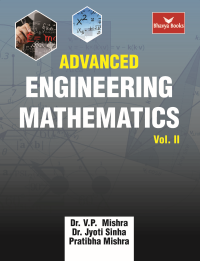•## Advanced Engineering Mathematics Vol. II (Bhavya Books)

In Stock
ISBN 978-93-83992-41-6 Dr. V.P. Mishra, Dr. Jyoti Sinha, Pratibha Mishra Bhavya Books 1st 2019 2019 525 Print In Stock
Rs 495.00Rs 446.00

#### CONTENTS

• Partial Differential Equations and Solution to First Order Equations • Laplace Transformation and its Inverse, Convolution, Applications of L’Transform in Solving Differential Equations • Partial Differential Equations of Higher Order and Applications • Fourier Transforms and their Applications • Difference Equations, Z-transform and Solution to Difference Equations by Z-transform • Numerical Solution to Equations in One Variable and Simultaneous Equations • Finite Differences, Divided Differences and Interpolation • Numerical Differentiation and Numerical Integration • Numerical Solution to Ordinary Differential Equations of Order One and Order Two • Numerical Solution to Partial Differential Equations • Theory of Sets, Relations and Functions, Partial and Total Order Relation, Lattice, Boolean Algebra • Mathematical Logic, Counting Principles and Recurrence Relations • Algebraic Structures • Graph Theory • Answers and Hints to the Problems in Exercises.

• Author : Dr. V.P. Mishra, Dr. Jyoti Sinha, Pratibha Mishra
• Publisher : Bhavya Books
• Edition : 1st 2019
• Publishing Year : 2019
• Total Pages : 525